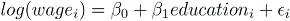Want to share your content on R-bloggers? click here if you have a blog, or here if you don't.One of the most frequently encountered issues in econometrics is endogeneity.
Consider the simple Ordinary Least Squares (OLS) regression setting in which we model wages as a function of years of schooling (education):One of the main assumption of OLS is that the independent variables are not correlated with the error term. However, this is seldom the case in most practical applications. The violation of this assumption causes the beta estimates to be biased. In the above example, years of schooling (education) is likely to be correlated with ‘individual ability’ which is part of the error term. Therefore, estimating this model would give a biased estimate of the beta parameter of education as it is considered endogenous. One option is to obtain a measure of ability such as IQ and have it as an independent variable in the model. Availability of such data is not always possible.

A frequently used approach is the method of instrumental variables: intuitively, we need to identify a variable (called instrumental variable) that is not part of the model, has high correlation with the endogenous variable and is uncorrelated with the error term. In our example, one possible candidate for instrumental variable (IV) is father’s education. The appropriateness of the IV is always debatable and it would be a good thought experiment to see why father’s education can fulfill the criteria for an IV we just defined and why it might fail.

The following exercises provide a basic introduction to using instrumental variable technique in R.

Answers to the exercises are available here.

Exercise 1
Load the AER package (package description: here). Next, load `PSID1976` dataset provided with the AER package. This has data regarding labor force participation of married women.
The data is sourced from: Mroz, T. A. (1987) The sensitivity of an empirical model of married women’s hours of work to economic and statistical assumptions. Econometrica 55, 765–799.

Exercise 2
Get summary statistics for the data and identify possible candidates as instrumental variables for education.

Exercise 3
Regress `log(wage)` on `education`. Why do you get an error message? (Hint: focus on the `participation` variable)

Exercise 4
Regress `log(wage)` on `education` and state the estimated return to another year of education for women that participated in the labor force. (Hint: use the `subset` command)

Note: the following exercises are based on the subsample of 428 working women used on exercise-4.

Exercise 5
Plot `log(wage)` against `education` along with the fitted regression line from exercise-4.

Exercise 6
Regress `education` on `feducation` (father’s years of education) and comment on the estimated correlation.

Exercise 7
The IV estimate of the return to education can be found using the two-stage least squares (2SLS) procedure.
Regress `log(wage)` on the fitted values of the model estimated in exercise-6.
The beta parameter for the fitted values is the IV estimate for return to education.
Note that the associated standard errors reported are incorrect as these are not based on the usual OLS formula.

Exercise 8
The `ivreg` function provides a convenient way to estimate IV models and get correct standard errors.
Using the `ivreg` function, regress `log(wage)` on education and specify `feducation` as the IV.
Do you get the same beta estimates as in exercise-6?

Exercise 9
Get 90% confidence intervals for the return to education from exercises 4 and 8.

Exercise 10
Repeat exercises 6 and 8 using mother’s education (`meducation`) as the IV and comment on the results.